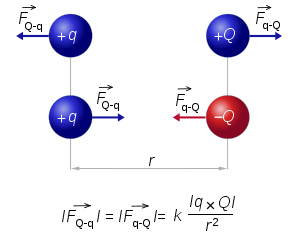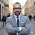# Force-Directed graph layout approximation part II've been working on these last days in a Force-Directed graph layout approximation applicated in my VLO Framework (That's why I've been off these days without posting anything). The system consists on applying physics laws to each node calculating the repulsion force using Coulomb's law and the attractive force using Hooke's law. To do this, I've made a lot of changes to accomplish all the requirements of the new functionality. Now, every TBox class implements a TNode class which has all the physics components like hooke_force, coulomb_force, speed, mass, etc. And the manager ThundaxBoxManager that handle all the canvas methods now implements the TGraph class which has all the data about repulsion calculation, attraction calculation, energy of the system, etc. The algorithm is easy to understand because it works in a simple way. We need to iterate every node and calculate in steps the hooke and coulomb force for everyone. In every step there will be new forces that interact with each node, and when the total amount of energy is nearly zero, we can stop the algorithm.

I've been searching on the internet different approximations in many programming languages, but I've only found it in javascript, perl and python. My approximation is done with Delphi, and I've made a win32 desktop application to show you this:
You only need to run the program, create the different nodes, connect them with edges and then play the force-directed layout algorithm. I'm still refactoring some parts of the code and I think I'll release another build in a few days.

I've also made some videos that show the running of the algorithm:

In this videos you can see different representations of graphs and it's new representation after applying the force-directed layout. With this algorithm we make the graph more human readable and understandable. If we take a look on the second video, this shows you that if we put more than one graph, the system is capable to show you all of them separately applying physic's laws.

• The algorithm:
The algorithm consists on calculating the different forces that every node as a particle has and continue with this until a level of kinetic energy is reached. The skeleton of the algorithm looks like this:

`while (not calculation_finished)total_kinetic_energy := 0for i := 0 to Boxlist.Count - 1 dobegin    node := Boxlist.items[i];    node.CoulombForce.setVal(0, 0);    for j := 0 to Boxlist.Count - 1 do    begin        other_node := Boxlist.items[j];        node.CoulombForce := node.CoulombForce + Coulomb_repulsion( node, other_node )    end;    for k := 0 to Boxlist.Count - 1 do    begin        node := Boxlist.items[i];        node.hookeForce.setVal(0, 0);        for l := 0 to High(node.Neighbour) do        begin          other_node := node.Neighbour[l];          node.hookeForce := node.hookeForce + Hooke_attraction( node, other_node )        end;    end;    node.speed.x := node.speed.x + (node.CoulombForce.X + node.hookeForce.X));    node.speed.y := node.speed.y + (node.CoulombForce.Y + node.hookeForce.Y));    total_kinetic_energy := total_kinetic_energy + node.mass * sqr(node.velocity);end;if total_kinetic_energy < Epsilon then   calculation_finished := true;end;`

In this piece of code (I'll describe this better in the next articles) we can see the easy calculation of the system. We only need to add some properties to the classes and calculate correctly the different forces (here is the big problem).

For the next days I'm preparing different articles showing this interesting approximation going through the different classes, and news features that I've had to build to improve the performance of the GUI. I hope you find interesting this, and don't hesitate to leave any comment on the post.

1.Nice job. i'm trying to work on a similar project, but with MATLAB and the input will specified in form of an adjacency matrix. i dont know if u could be of help. thanks.

2.Hi Dammy,

Check out Matlab Central. There they have loads of Matlab examples, and when I do something with it I always go there. Here is an interesting link :
Problem with biograph layout

1.can you elaborate a little bit on how you come to the new x and y positions of the nodes?

thanks

2.Hi Perky,

Check out wikipedia as it is very well explained. Force-Based algorithms. Each node has x,y position and dx,dy velocity and mass m. There is usually a spring constant, s, and damping: 0 < damping < 1. The force toward and away from nodes is calculated according to Hooke's Law and Coulomb's law.